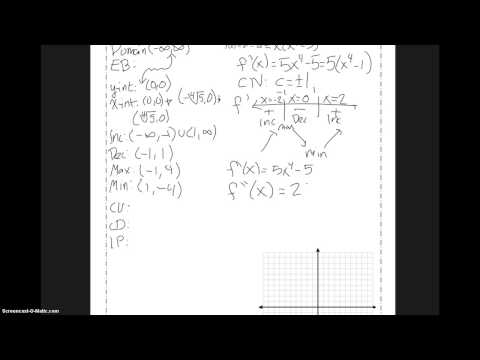### 3.6 Day 2 Example 2# 3.6 Day 2 Example 2

## 16 Video Interactions

1. 00:00:23

What is always the domain of a polynomial function?

• Positive Numbers
• All Real Numbers
• Imaginary Numbers
• Natural Numbers

2. 00:00:35

What is the end behavior for this function?

• Rising, left to right
• Falling, left to right
• Pointing upwards on both sides
• Pointing downwards on both sides

3. 00:00:49

What is the y-intercept for this function?

• (0, 5)
• (0, 0)
• (0, -5)
• (-5, 0)

4. 00:01:45

When do you use the plus or minus in front of a radical?

• Even powers
• Odd Powers
• Never

5. 00:02:10

What is the derivative, f'(x)?

• f'(x) = x^5 - 5
• f'(x) = 5x^4 - 5
• f'(x) = 5x^4
• 5x^4 - 5x

6. 00:02:30

What are the critical numbers?

• c= 0
• c=1
• c= - 1
• c= 4
• c= 5
• c = -5
• c= -4

7. 00:03:16

What happens in the original graph when the derivative changes from positive to negative?

• Minimum
• Maximum

8. 00:03:50

What is the y-value of the maximum that occurs at x = -1?

• y = 4
• y = -4
• y = 0

9. 00:04:01

What is the y-value of the minimum that occurs at x = 1?

• y = 0
• y = -4
• y = 4

10. 00:04:22

What is an inflection point?

• Where the function changes concavity
• Extrema
• Where the function changes sign
• Where the function is increasing

11. 00:04:35

What is the second derivative, f''(x)?

• f''(x) = 5x^3
• f''(x) = 20x^3
• f''(x) = 20x^3 - 5
• f''(x) = 5x^3 - 5

12. 00:04:45

What is the fence post for the second derivative test?

• -1
• 20

13. 00:04:58

When the second derivative is negative, what does that tell you about the original function?

• Increasing
• Decreasing
• Concave Up
• Concave Down

14. 00:05:07

When the second derivative is positive, what does that tell you about the original function?

• Increasing
• Decreasing
• Concave Up
• Concave Down

15. 00:06:35

What does the graph look like when the function is concave down?

• Increasing
• Decreasing
• Facing Up
• Facing Down

16. 00:06:56

Now you are ready to try on your own. You have been performing first and second derivative tests for three weeks. Now you are sketching the graph in addition. Do not use your graphing calculator!

• No, I am probably going to use my graphing calculator and then regret that decision when I have a quiz/test where I am unable to use it
• Yes, I understand and will not use my graphing calculator

Click here to preview, use, or make a copy of this interactive video lesson plan (bulb).